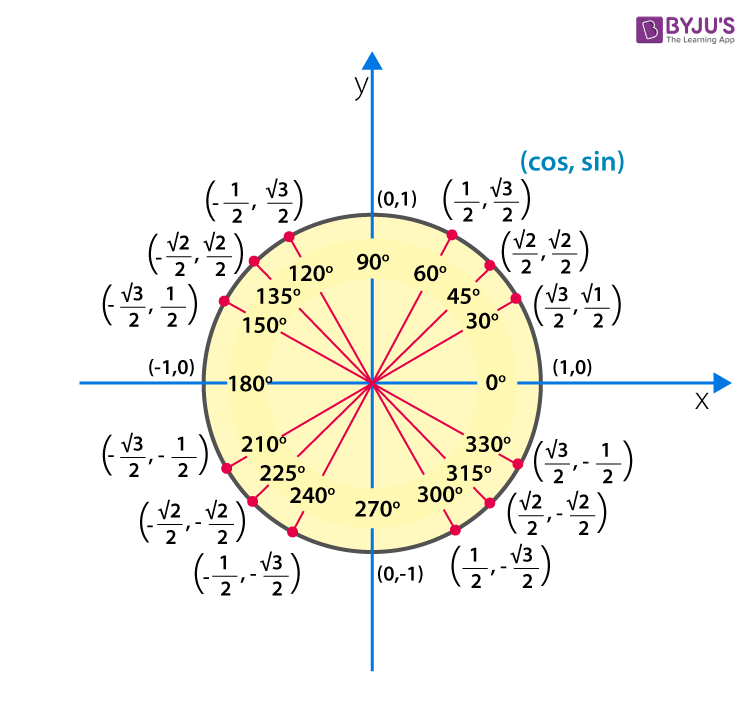How is cosine calculated?

Trigonometry is an important branch of mathematics that deals with finding the missing sides and angles of a right angled triangle. Trigonometry consists of different ratios, functions and identities. The six important trigonometry ratios are:

• Sine (sin)
• Cosine (cos)
• Tangent (tan)
• Cosecant (cosec)
• Secant (sec)
• Cotangent (cot)

All these ratios are related to each other in some ways. Here, cosec, sec and cot are the reciprocal of sin, cos and tan, respectively.

Suppose ABC is a triangle right angled at B, AC is the hypotenuse and AB and BC are the perpendicular and base, respectively. Now, the trigonometry ratios can be written as:

sin A = AB/AC = Perpendicular/Hypotenuse

cos A = BC/AC = Base/Hypotenuse

tan A = AB/BC = Perpendicular/Base = sin A/cos A

cosec A = Hypotenuse/Perpendicular = AC/AB = 1/sin A

sec A = Hypotenuse/Base = AC/BC = 1/cos A

cot A = Base/Perpendicular = BC/AB = 1/tan A = cos A/sin A

Thus, cosine of angle A is the ratio of the sides BC and AC, i.e. base and hypotenuse. Also, when any two sides of a right triangle are known, we can find the third side using Pythagoras theorem, if it is necessary to find the cosine value.The above figure shows the values of cosine and sine for different measures of angles. These can be tabulated as:

 Angle 0° 30° 45° 60° 90° 180° 270° 360° sin θ 0 1/2 1/√2 √3/2 1 0 -1 0 cos θ 1 √3/2 1/√2 1/2 0 -1 0 1

These are values of cosine for the basic angles which we use frequently while solving various problems in maths. Click here to learn more about trigonometry and related concepts.(1)(0)